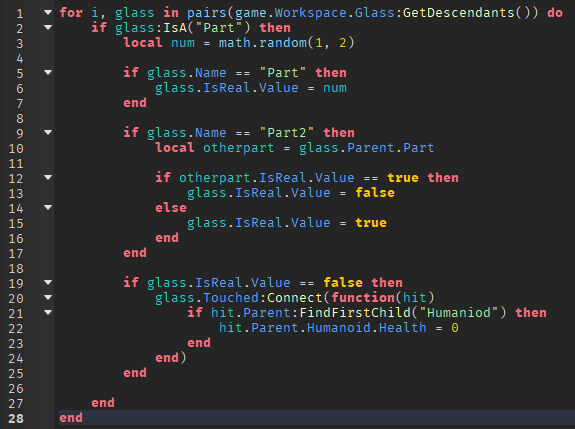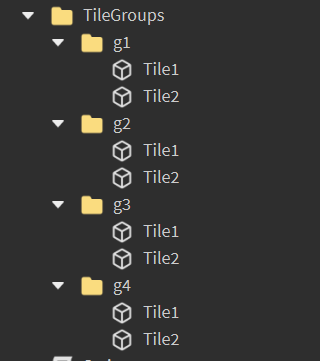# Setting a 50 / 50 Chance on a Pair of Objects

Hi, I want to make a glass bridge game (you know like from squid game), but whenever I try to make one object in a pair of glass break, it doesn’t do so. They could both be correct (which I don’t want), both wrong (which I also don’t want), or just normal. I don’t know what to do. I think my script would be pointless because the methods I use I think are depracated or not necessary. Thanks!

“Both wrong”. But if both tiles are wrong then how will players progress, is this what you want?

Sorry, I don’t want them to be both wrong. I want only one to be right.

1 Like

You can use a `math.random` function.

For example:

``````local random = math.random(1, 2)

if random == 1 then
--  Make the part break script here..
else
-- Make the part normal script here..
end
``````

This is a simple example on how to use `math.random`. You can check more at this Roblox Documentation.

aaaaa

Can you provide us the code you have tried so far?

Have you tried doing something like this:

``````local glass = {
script.Parent:FindFirstChild("glass1").Name,
script.Parent:FindFirstChild("glass2").Name
}

local random = glass[math.random(1, #glass)]
if random then
-- script.Parent:FindFirstChild(random).CanCollide = false
-- break script
end
``````The result of this code is all of them being correct

I noticed that you misspelt humanoid

Well one thing I noticed is that in line 21.

``````if hit.Parent:FindFirstChild("Humaniod") then
``````

which is not vaild.

Change it to:

``````  if hit.Parent:FindFirstChild("Humanoid") then
``````

Other than that the scripts looks correct; so it should have no errors. Maybe it didn’t work due to `Humanoid` being spelt wrong so even if the glass was Touched, it couldn’t kill the user who touched it.

Still not working. IsReal is not being applied anywhere. (IsReal is a boolvalue; true = wont break, false = will break)

You could try doing something like this so you can avoid using booleans:

``````local glassTable = {}

for i, glass in pairs(script.Parent:GetDescendants()) do
if glass:IsA("Part") then
table.insert(glassTable, glass)
end
end

local random = glassTable[math.random(1, #glassTable)]

random.Touched:Connect(function(hit)
if hit.Parent:FindFirstChild("Humanoid") then
hit.Parent.Humanoid.Health = 0
end
end)
``````
1 Like

Yes I could do that, but I don’t want to put that script in every paired model. I want to put it in server script service.

Group the tiles in pairs. This is example for cancollide but you’d just continue and do your glass breaking instead.``````local tileGroups = workspace.TileGroups
for i,v in ipairs(tileGroups:GetChildren()) do
v:GetChildren()[math.random(1,2)].CanCollide = false
end
``````
3 Likes

Would you have to do `v:GetChildren()["Tile"..math.random(1,2)].CanCollide = false` instead because you have the word tile before the number.

No. v:GetChildren() creates a table of two parts. So v:GetChildren() is the same as {Tile1,Tile2}
By doing v:GetChildren()[math.random(1,2)], it either references the first part or the second part in the table

1 Like

This topic was automatically closed 14 days after the last reply. New replies are no longer allowed.